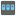• # Searching for digits

## Question related to missionHouse Password

So I figured out how to do the process with the re module, however prior to that I was trying to do it without any import. I was able to match upper and lower case letters, but I could not find a built in function to detect numbers. Any ideas?

def check(x): capital = 0 lower = 0 digit = 0

```if len(x) > 9:
for c in x:
if c == c.upper():
capital += 1
elif c == c.lower():
lower += 1
elif c == c.digit():
digit += 1
elif capital >= 1 and lower >= 1 and digit >= 1:
return True or False
```

check(x)8